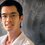# Polynomial problem

Let $g(x)$ be a polynomial such that $g(x)=x^{8}-4x^{7}+7x^{6}+ax^{5}+bx^{4}+cx^{3}+dx^{2}+ex+f$ factorizes into $8$ linear factors.Also roots of the polynomial are all positive,where $a,b,c,d,e,f$ are all real numbers.Sum of all possible values of $f$ can be written as $\frac{a}{b}$,where $a$ and $b$ are co-prime positive integers.Find $a+b?$ I submitted it to brilliant but they rejected,no problem.So you must try it.I want to know multiple methods of this problem.Thank You.After 2 solutions are submitted I will also share my awesome method....!Note by Kishan K
6 years, 5 months ago

This discussion board is a place to discuss our Daily Challenges and the math and science related to those challenges. Explanations are more than just a solution — they should explain the steps and thinking strategies that you used to obtain the solution. Comments should further the discussion of math and science.

When posting on Brilliant:

• Use the emojis to react to an explanation, whether you're congratulating a job well done , or just really confused .
• Ask specific questions about the challenge or the steps in somebody's explanation. Well-posed questions can add a lot to the discussion, but posting "I don't understand!" doesn't help anyone.
• Try to contribute something new to the discussion, whether it is an extension, generalization or other idea related to the challenge.

MarkdownAppears as
*italics* or _italics_ italics
**bold** or __bold__ bold
- bulleted- list
• bulleted
• list
1. numbered2. list
1. numbered
2. list
Note: you must add a full line of space before and after lists for them to show up correctly
paragraph 1paragraph 2

paragraph 1

paragraph 2

[example link](https://brilliant.org)example link
> This is a quote
This is a quote
    # I indented these lines
# 4 spaces, and now they show
# up as a code block.

print "hello world"
# I indented these lines
# 4 spaces, and now they show
# up as a code block.

print "hello world"
MathAppears as
Remember to wrap math in $$ ... $$ or $ ... $ to ensure proper formatting.
2 \times 3 $2 \times 3$
2^{34} $2^{34}$
a_{i-1} $a_{i-1}$
\frac{2}{3} $\frac{2}{3}$
\sqrt{2} $\sqrt{2}$
\sum_{i=1}^3 $\sum_{i=1}^3$
\sin \theta $\sin \theta$
\boxed{123} $\boxed{123}$

Sort by:

Perhaps they rejected it because you use $a,b$ for the coefficients of $g(x)$ as well as the value of $f$.

By Vieta's Relations, the sum of the $8$ roots is $4$ and the sum of the squares is $4^2 - 2\cdot 7 = 2$.

Hence, the arithmetic mean of the roots is $\dfrac{4}{8} = \dfrac{1}{2}$ and the root-mean-square of the roots is $\sqrt{\dfrac{2}{8}} = \dfrac{1}{2}$.

Therefore, we have the equality case of the RMS-AM inequality. So, all the roots are equal to $\dfrac{1}{2}$.

Thus, $g(x) = \left(x - \tfrac{1}{2}\right)^8$ is the unique polynomial $g(x)$. So, $f = g(0) = \dfrac{1}{2^8} = \dfrac{1}{256} \leadsto a+b = \boxed{257}$.

- 6 years, 5 months ago

I did the same but I used Cauchy Schwarz Inequality instead of RMS-AM inequality.But nice solution.

- 6 years, 5 months ago

I would like to know why you consider that inequality? I mean you get AM=RMS but where does it really help?

Thanks!

- 6 years, 5 months ago

The RMS-AM Inequality states that if $x_1, \ldots, x_n \ge 0$, then $\sqrt{\dfrac{1}{n}\displaystyle\sum_{k = 1}^{n}x^2_k} \ge \dfrac{1}{n}\displaystyle\sum_{k=1}^{n}x_k$.

Furthermore, $\sqrt{\dfrac{1}{n}\displaystyle\sum_{k = 1}^{n}x^2_k} = \dfrac{1}{n}\displaystyle\sum_{k=1}^{n}x_k = a$ if and only if $x_1 = \cdots = x_n = a$.

In this problem, that theorem allows us to conclude that the roots of $g(x)$ are all equal to $\tfrac{1}{2}$.

This shows that there is only one possible polynomial $g(x)$, namely $g(x) = \left(x - \tfrac{1}{2}\right)^8$.

- 6 years, 5 months ago

Awesome as always, thanks Jimmy! :)

Mind throwing some light on how you instantly came up with that inequality?

- 6 years, 5 months ago